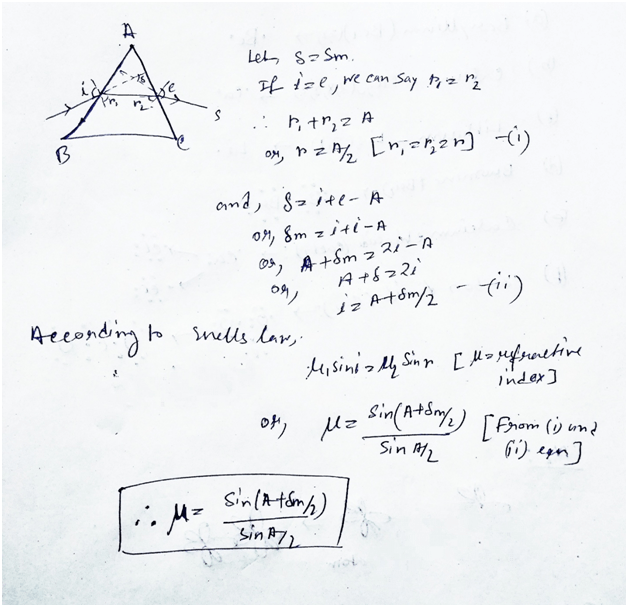# Telangana SCERT Class 10 Physical Science Chapter 5 Human Eye and Colourful World Solution

Telangana SCERT Class 10 Physical Science Chapter 5 Solution – Human Eye and Colourful World. Here in this post we provides Class 10 Physical Science Human Eye and Colourful World Telangana State Board Solution.  Telangana State Board English Class X Medium Students can download this Solution to Solve out Improve Your Learning Questions and Answers.

## Telangana State Board Class 10 Physical Science Chapter 5 Human Eye and Colourful World Solution:

### Human Eye and Colourful World

Exercise: –

Reflections on Concepts

1) How do you correct the eye defect Myopia?

Ans: – The detect myopia can be correct by using the concave lens.

2) Explain the correction of the eye defect Hypermetropia.

Ans: – The Hypermetropia is the disease in which we couldn’t see clearly the object which is placed closely to us. This problem can be correct by the uses of the convex lens.

3) How do you find experimentally the refractive index of material of a prism?

Ans: –4) Explain the formation of rainbow.

Ans: – The rainbow is nothing but the dispersion of the light rays. In the rainy day the small droplets of water act as the little prism. This the rays coming from the sun fallen in that water droplets and these rays are divided into seven colours which forms the rainbow.

5) Explain two activities for the formation of artificial rainbow. (AS3).

Ans: – The activity for the formation of the artificial rainbow is –

(i) When the sun rays are falling on a white wall if we make the flow of water by restricting the most of volume of water from pipe then we see the rainbow is formed by the flowing of water.

(ii) In a bekar after filling with the water if we placed white board on it then we will see that the shadows of this on the wall which is the rainbow-like phenomenon.

6) Light of wavelength λ1 enters a medium with refractive index n2 from a medium with refractive index n1. What is the wave length of light in second medium?

Ans: – As we all know that the refractive index of a medium is the ratio of speed in air to the wavelength or retroactive index=(speed in air / wavelength).

As the speed is same so the wavelength will be y1n1/n2.

7) Why does the sky sometimes appear white?

Ans: – The sky appears white sometimes because of the scattering of light from the different particles present in air. The sunlight ray failing on different particle in air and scattered by which we see it white.

8) A person is viewing an extended object. If a converging lens is placed in front of his eye, will he feel that the size of object has increased? Why?

Ans: – The converging lens is nothing but the convex lens this lens always forms an image wider from the previous one. So, in this as there is using a convex lens the person views an increased in size of an object.

Application of Concepts

1) Incident ray on one of the faces (AB) of a prism and emergent ray from the face AC is given in figure -1. Complete the ray diagram.

Ans: –2) Glass is known to be a transparent material. But ground glass is opaque and white in colour. Why?

Ans: – The normal glasses which are being used most in the households are transfers the light rays through it. But the ground glass is opaque and white because it doesn’t flow the light rays from it.

3) A light ray falls on one of the faces of a prism at an angle 40°So that it suffers angle of minimum deviation of 30°. Find the angle of prism and angle of refraction at the given surface.

Ans: –4) The focal length of a lens suggested to a person with Hypermetropia is 100cm. Find the distance of near point and power of the lens.

Ans: – As we all know that, 1/f=1/v-1/u;

Here, f=100cm, v=25cm (we assume near point as 25cm).

So, 1/u= 1/100 – 1/25;

Or, u=33.3cm.

And the power is,

P=1/focal length

= 100/100=1D.

Multiple choice questions

1) The size of an object as perceived by an eye depends primarily on

A) actual size of the object B) distance of the object from the eye C) aperture of the pupil D) size if the image formed on the retina

Ans: – option (D).

2) When objects at different distances are seen by the eye which of the following remain constant?

A) focal length of eye-lens B) object distance from eye-lens  C) the radii of curvature of eye-lens D) image distance from eye-lens.

Ans: – option (A).

3) During refraction, ________ will not change.

A) Wave length B) frequency C) speed of light D) all the above.

Ans: – option (c).

4) A ray of light falls on one of the lateral surfaces of an equilateral glass prism placed on the horizontal surface of a table as shown in fig.2.For minimum deviation of ray, which of the following is true?

A) PQ is horizontal B) QR is horizontal C) RS is horizontal D) either PQ or RS is horizontal.

Ans: – option (B).

5) Far point of a person is 5m. In order that he has normal vision what kind of spectacles should he use

A) Concave lense with focal length 5m

B) concave lense with focal length 10m

C) Convex lense with focal length 5m

D) convex lense with focal length 2.5m.

Ans: – option (A).

6) The process of re-emission of absorbed light in all directions with different intensities by the atom or molecule is called –

A) Scattering of light B) dispersion of light C) reflection of light D) refraction of light.

Ans: – option (A) scattering of light.

Updated: January 19, 2022 — 1:10 pm